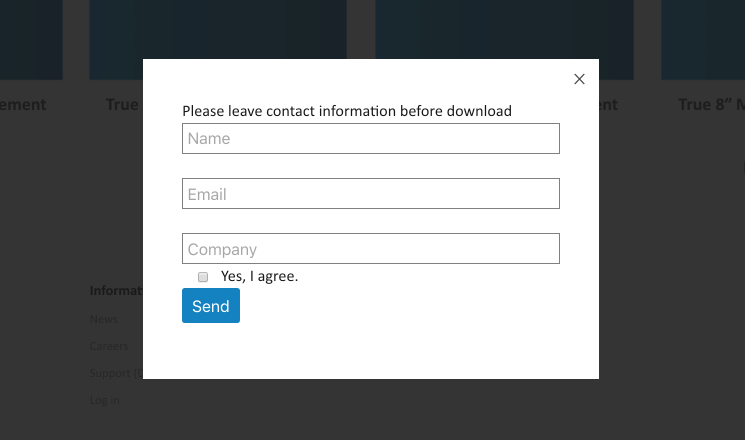``````document.addEventListener('wpcf7mailsent', function(event) {
location = '下載的檔案連結';
}, false);````````````function mxp_wpcf7_do_shortcode() {
//給CF7啟用短碼機制
}

``````function mxp_ajax_get_download_form(\$request) {
if (!wp_verify_nonce(\$nonce, 'wp_rest')) {
wp_send_json_error(array('code' => 500, 'data' => '', 'msg' => '錯誤的請求'));
}
wp_send_json_success(array('code' => 200, 'data' => do_shortcode('[contact-form-7 404 "找不到符合條件的項目"]')));
}
'methods' => 'GET',
));
});``````

``````\$('div.wpcf7 > form').each(function() {
var \$form = \$(this);
wpcf7.initForm(\$form);
if (wpcf7.cached）{
wpcf7.refill(\$form);
}
});``````

``````// 建立cookie
function ks_createCookie(name, value, days, path) {
if (days) {
var date = new Date();
date.setTime(date.getTime() + (days * 24 * 60 * 60 * 1000));
var expires = "; expires=" + date.toGMTString();
} else var expires = "";
document.cookie = name + "=" + value + expires + "; path=/";
}
//讀取
var nameEQ = name + "=";
for (var i = 0; i < ca.length; i++) {
var c = ca[i];
while (c.charAt(0) == ' ') c = c.substring(1, c.length);
if (c.indexOf(nameEQ) == 0) return c.substring(nameEQ.length, c.length);
}
return null;
}
//刪除
}``````

Share:

Filter